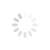## Tips and Resources# UnityEngine.Vector3 is not marked as Serializable

Guide for serializing Vector3 data in Unity.

When attempting to save a Vector3 variable's value (or a Quaternion for that matter) to disk, you may find that Unity will throw a UnityEngine.Vector3 is not marked as Serializable error. To work around this, you can create a new SerializedVector struct that can be used to serialize your Vector3 and saved to disk.

Create a new C# script in Unity called SerializedVector3 and enter the following code:

`using UnityEngine;[System.Serializable]public struct SerializedVector3 { public float x; public float y; public float z; public SerializedVector3(float x, float y, float z) {   this.x = x;   this.y = y;   this.z = z; } public SerializedVector3(Vector3 v) { this.x = v.x; this.y = v.y; this.z = v.z; } public override bool Equals(object obj) {   if ((obj is SerializedVector3) == false){   return false;   }   var s = (SerializedVector3)obj;   return x == s.x && y == s.y && z == s.z; } public override int GetHashCode() {   var hashCode = 373119288;   hashCode = hashCode * -1521134295 + x.GetHashCode();   hashCode = hashCode * -1521134295 + y.GetHashCode();   hashCode = hashCode * -1521134295 + z.GetHashCode();   return hashCode; } public Vector3 ToVector3() {   return new Vector3(x, y, z); } public static bool operator == (SerializedVector3 a, SerializedVector3 b) {   return a.x == b.x && a.y == b.y && a.z == b.z; } public static bool operator != (SerializedVector3 a, SerializedVector3 b) {   return a.x != b.x || a.y != b.y || a.z != b.z; } public static implicit operator Vector3(SerializedVector3 x) {   return new Vector3(x.x, x.y, x.z); } public static implicit operator SerializedVector3(Vector3 x) {   return new SerializedVector3(x.x, x.y, x.z); }}`

To use the serialized Vector3, call it's constructor passing in a Vector3, for example:

`...public Vector3 GetCheckPointPosition() { return checkpointPosition;  } checkpointPosition = new SerializedVector3(GetCheckPointPosition());`